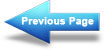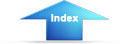Custom SearchConductanceMatter, Energy, and ElectricityChapter 1 SummaryWattage Rating

When a current is passed through a resistor, heat is developed within the resistor. The resistor must be capable of dissipating this heat into the surrounding air; otherwise, the temperature of the resistor rises causing a change in resistance, or possibly causing the resistor to burn out.

The ability of the resistor to dissipate heat depends upon the design of the resistor itself. This ability to dissipate heat depends on the amount of surface area which is exposed to the air. A resistor designed to dissipate a large amount of heat must therefore have a large physical size. The heat dissipating capability of a resistor is measured in WATTS (this unit will be explained later in chapter 3). Some of the more common wattage ratings of carbon resistors are: one-eighth watt, one-fourth watt, one-half watt, one watt, and two watts. In some of the newer state-of-the-art circuits of today, much smaller wattage resistors are used. Generally, the type that you will be able to physically work with are of the values given. The higher the wattage rating of the resistor the larger is the physical size. Resistors that dissipate very large amounts of power (watts) are usually wirewound resistors. Wirewound resistors with wattage ratings up to 50 watts are not uncommon. Figure 1-30 shows some resistors which have different wattage ratings. Notice the relative sizes of the resistors.Figure 1-30. - Resistors of different wattage ratings.

Standard Color Code System

In the standard color code system, four bands are painted on the resistor, as shown in figure 1-31.Figure 1-31. - Resistor color codes.

Examples of resistor color codes.

The color of the first band indicates the value of the first significant digit. The color of the second band indicates the value of the second significant digit. The third color band represents a decimal multiplier by which the first two digits must be multiplied to obtain the resistance value of the resistor. The colors for the bands and their corresponding values are shown in Table 1-1.

Table 1-1.Standard Color Code for Resistors

Use the example colors shown in figure 1-31.Since red is the color of the first band, the first significant digit is 2. The second band is violet, therefore the second significant digit is 7. The third band is orange, which indicates that the number formed as a result of reading the first two bands is multiplied by 1000. In this case 27 1000 = 27,000 ohms. The last band on the resistor indicates the tolerance; that is, the manufacturer's allowable ohmic deviation above and below the numerical value indicated by the resistor's color code. In this example, the color silver indicates a tolerance of 10 percent. In other words, the actual value of the resistor may fall somewhere within 10 percent above and 10 percent below the value indicated by the color code. This resistor has an indicated value of 27,000 ohms. Its tolerance is 10 percent 27,000 ohms, or 2,700 ohms. Therefore, the resistor's actual value is somewhere between 24,300 ohms and 29,700 ohms.

When measuring resistors, you will find situations in which the quantities to be measured may be extremely large, and the resulting number using the basic unit, the ohm, may prove too cumbersome. Therefore, a metric system prefix is usually attached to the basic unit of measurement to provide a more manageable unit. Two of the most commonly used prefixes are kilo and mega. Kilo is the prefix used to represent thousand and is abbreviated k. Mega is the prefix used to represent million and is abbreviated M.

In the example given above, the 27,000-ohm resistor could have been written as 27 kilohms or 27 kW. Other examples are: 1,000 ohms = 1 kW; 10,000 ohms = 10 kW; 100,000 ohms = 100 kW. Likewise, 1,000,000 ohms is written as 1 megohm or 1 MW and 10,000,000 ohms = 10 MW.

Q62.A carbon resistor has a resistance of 50 ohms, and a tolerance of 5 percent. What are the colors of bands one, two, three, and four, respectively?SIMPLIFYING THE COLOR CODE. - Resistors are the most common components used in electronics. The technician must identify, select, check, remove, and replace resistors. Resistors and resistor circuits are usually the easiest branches of electronics to understand.

The resistor color code sometimes presents problems to a technician. It really should not, because once the resistor color code is learned, you should remember it for the rest of your life.

Black, brown, red, orange, yellow, green, blue, violet, gray, white - this is the order of colors you should know automatically. There is a memory aid that will help you remember the code in its proper order. Each word starts with the first letter of the colors. If you match it up with the color code, you will not forget the code.

Bad Boys Run Over Yellow Gardenias Behind Victory Garden Walls,
or:

 Black Bad Brown Boys Red Run Orange Over Yellow Yellow Green Gardenias Blue Behind Violet Victory Gray Garden White Walls

There are many other memory aid sentences that you might want to ask about from experienced technicians. You might find one of the other sentences easier to remember.

There is still a good chance that you will make a mistake on a resistor's color band. Most technicians do at one time or another. If you make a mistake on the first two significant colors, it usually is not too serious. If you make a miscue on the third band, you are in trouble, because the value is going to be at least 10 times too high or too low. Some important points to remember about the third band are:

When the third band is . . . .

Black, the resistor's value is less than 100 ohms.
Brown, the resistor's value is in hundreds of ohms.
Red, the resistor's value is in thousands of ohms.
Orange, the resistor's value is in tens of thousands of ohms.
Yellow, the resistor's value is in hundreds of thousands of ohms.
Green, the resistor's value is in megohms.
Blue, the resistor's value is in tens of megohms or more.

Although you may find any of the above colors in the third band, red, orange, and yellow are the most common. In some cases, the third band will be silver or gold. You multiply the first two bands by 0.01 if it is silver, and 0.1 if it is gold.
The fourth band, which is the tolerance band, usually does not present too much of a problem. If there is no fourth band, the resistor has a 20-percent tolerance; a silver fourth band indicates a 10-percent tolerance; and a gold fourth band indicates a 5-percent tolerance. Resistors that conform to military specifications have a fifth band. The fifth band indicates the reliability level per 1,000 hours of operation as follows:

 Fifth band color Level Brown 1.0% Red 0.1% Orange 0.01% Yellow 0.001%

For a resistor whose the fifth band is color coded brown, the resistor's chance of failure will not exceed 1 percent for every 1,000 hours of operation.

In equipment such as the Navy's complex computers, the reliability level is very significant. For example, in a piece of equipment containing 10,000 orange fifth-band resistors, no more than one resistor will fail during 1,000 hours of operation. This is very good reliability. More information on resistors is contained in NEETS Module 19.

Q63.A carbon resistor has the following color bands: The first band is yellow, followed by violet, yellow, and silver. What is the ohmic value of the resistor?Q64.The same resistor mentioned in question 63 has a yellow fifth band. What does this signify?Q65.A resistor is handed to you for identification with the following color code: the first band is blue, followed by gray, green, gold, and brown. What is the resistor's value?Some resistors, both wirewound and composition, will not use the resistor color code. These resistors will have the ohmic value and tolerance imprinted on the resistor itself.Integrated Publishing, Inc. - A (SDVOSB) Service Disabled Veteran Owned Small Business